### Home > CALC > Chapter 1 > Lesson 1.2.2 > Problem1-40

1-40.
1. State the domain for each of the functions below. Homework Help ✎

1.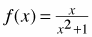2.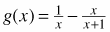3.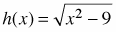4.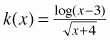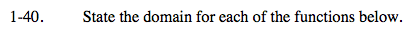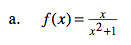We all know that 0 is excluded from the denominator of a fraction. What value of x, if any, would make the denominator x2 + 1 = 0?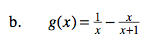Consider the domain of each part of the subtraction problem separately.

$\text{Since }g(x) \text{ is the difference between }\frac{1}{x} \text{ and }\frac{x}{x+1}, \text{ both excluded values must be excluded from } g(x).$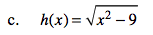Typically, a square root function has a domain of x ≥ 0, but in this case, x is squared... meaning both positive or negative values will yield positive outputs. However, do not neglect to consider the − 9.
What values of x, both positive and negative, will make x2 − 9 > 0?

x ≤ − 3 U x ≥ 3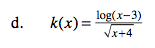Consider the domain of the numerator and the domain of the denominator separately.

I. What is the domain of log(x − 3)?

III. What value is excluded from the denominator of all fractions?

IV. Now combine these results to find the domain of k(x).

I. x > 3
II. x ≥ −4
III. x ≠ −4
IV. x > 3